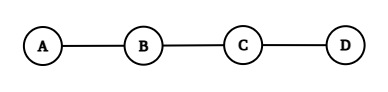# Morning Walk

Posted: 2 Jul, 2021
Difficulty: Moderate

## PROBLEM STATEMENT

#### You will output a string containing:

``````“Never” if they never meet.
“Point” if they meet at a point.
“Intersect” if they intersect each other.
``````

#### For Example:

``````For A = 1, B = 3, C = 5, D = 10
````````````They will never meet as their paths have no common points.
``````
##### Input Format:
``````The first line contains a single integer ‘T’ denoting the number of test cases to be run. Then the test cases follow.
The first line of each test case contains four space-separated integers representing A, B, C, D.
``````
##### Output Format:
``````For each test case, print a string according to the above conditions.
Output for each test case will be printed in a separate line.
``````
##### Note:
``````You are not required to print anything, it has already been taken care of. Just implement the function.
``````
##### Constraints:
``````1 <= T <= 10^4
-10^6 <= A, B, C, D <= 10^9

Time Limit: 1 sec.
``````Approach 1

Consider the paths are in the x-axis. Let us modify the given input such that the starting points of both the person are smaller than their corresponding ending points.

Now if the minimum value of the ending points is greater than the maximum value of starting points, then the paths will be intersecting.

Algorithm:

• Let ‘AlexStart’, ‘AlexEnd’, ‘BobStart’, ‘BobEnd’ are the modified values of the given input.
• ‘AlexStart’ will be the minimum of A and B, and ‘AlexEnd’ will be the maximum of them.
• ‘BobStart’ will be the minimum of C and D, and ‘BobEnd’ will be the maximum of them.
• Let ‘END’ be the minimum of both the ending points and ‘START’ be the maximum of both the starting points.
• If they are equal then the output will be “Point”.
• Else if ‘END’ is greater than ‘START’, then the output will be “Intersecting”.
• Else, the output will be “Never”.